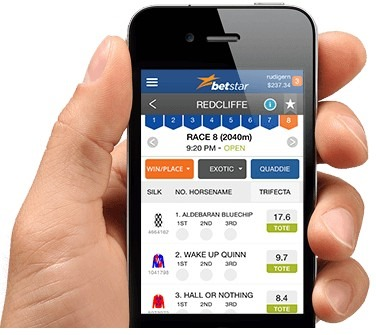Search by category:# Online Spread Betting for Dummies

Spread betting is a type of derivative financial product in that it has no value in and of itself and only achieves value if an underlying assets value changes. Whether the value is positive or negative will depend on the whether the trader decides to go long (buy) or go short (sell).

The basics of spread betting involve a trader speculating on whether the price of a specific asset will rise or fall. The asset can be an indie, commodity, share or currency pair and there are thousands to choose from. Now, if the trader believes the price of an asset will fall, they would sell (go short) at a specific amount per point fall in price.

So that if the asset was a share and it was currently valued at 100p, the trader would go short, for example, at 10 per point. If the share then dropped in price to 85p it would equal a 15 point drop x 10 = 150 profit. The only price to pay for a spread bet trade is the Spread price.

Another point is that the value of the spread bet price-per-point has nothing to do with the actual price of the base asset. A share price could be 10p but the trader went long at 100 per point and would earn 400 if the share price rises to 14p.

If one were to throw caution to the wind and bet 1000 on a price to rise from 2p to 3p and it did, then 1000 would be what you would earn. Similarly, and less attractive to note, the loss would be the same 1000 if the share actually dropped to 1p.

A Spread is a difference between the BID and ASK price of a trade. Brokers price Spread Bet shares so that if the actual asset or share price is 100p, the SELL (ASK) and BUY (BID) price would be something like 99p and 101p respectively. In this case, the Spread would be 2p and represents the fixed fee charged by the broker.

In the initial example, the actual profit would be 150 â€“ 10 = 140.

### Leverage and Margin

One of the chief advantages of Spread Betting is that it is a leveraged product so the full price of the trade does not need to be paid upfront. For example, a conventional trade requiring an investor to pay 1000 for 10,000 10p shares, would earn them 200 if the share price increased by 2p.

However, this would have required an initial outlay ofÂ  1000 plus the resulting commissions and taxes on the profit.

The small deposit or margin required for a Spread Betting trade is calculated in the following way assuming that the margin requirement for the trade was set to 5% (which is quite typical), they were waging 10 per Point and a share value of 100p:

Price/Point x Asset Price x 5% = 10 x 100 x 5% = 50

The 50 margins or deposit will be the same whether the profit was 150 or 1500.